Courses

# Test: Sets- 1

## 8 Questions MCQ Test Quantitative Aptitude for GMAT | Test: Sets- 1

Description
This mock test of Test: Sets- 1 for GMAT helps you for every GMAT entrance exam. This contains 8 Multiple Choice Questions for GMAT Test: Sets- 1 (mcq) to study with solutions a complete question bank. The solved questions answers in this Test: Sets- 1 quiz give you a good mix of easy questions and tough questions. GMAT students definitely take this Test: Sets- 1 exercise for a better result in the exam. You can find other Test: Sets- 1 extra questions, long questions & short questions for GMAT on EduRev as well by searching above.
QUESTION: 1

### Out of the 150 students of School X, 40 students have opted for only French as an extra subject. Some students have opted for German as an extra subject and 30 students have opted neither for German nor French. What is the total number of students who have opted for only German? (1) The total number of students opting for at least one subject out of German and French is 120.  (2) The number of students who opted for both German and French is one-third of the number of students who opted for neither of the two subjects.

Solution:

Steps 1 & 2: Understand Question and Draw Inferences

We are given that there are 150 students in a school. Out of these 150 students, 40 have opted only for French as an extra subject.

There are 30 students who haven’t opted for any of German or French as the extra subject.

Let’s say:

X = Number of students who opted for both the subjects

Y = Number of students who opted only for German as the extra subject

So, the above information can be represented as follows: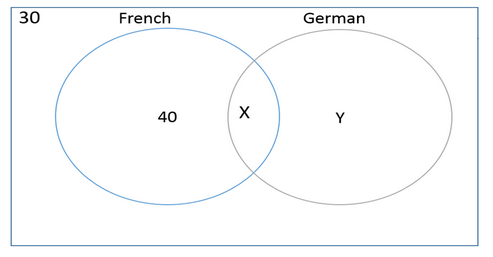The sum of the numbers in the four zones of the Venn diagram will be equal to the total number of students.

Thus,

40 + X + Y + 30 = 150

We get:

X + Y = 80      ……….. (1)

The question asks us to find the value of Y.

Step 3: Analyze Statement 1

The total number of students opting for at least one subject out of German and French is 120

This means,

40 + X + Y = 120

Or, X + Y = 80

As shown in equation (1), we already have this information. However, since we don’t have the value of X, we can’t find the value of Y.

Hence, Statement 1 is not sufficient to answer the question: What is the value of Y?

Step 4: Analyze Statement 2

The number of students who opted for both German and French is one-third of the number of students who opted for neither of the two subjects

Per this statement,

X=1/3(30)=10

By plugging in the value of X in Equation (1), we get,

Y = 70

Hence, Statement 2 alone is sufficient to answer the question: What is the value of Y?

Step 5: Analyze Both Statements Together (if needed)

Since Statement 2 alone is sufficient to answer the question, we don’t need to perform this step.

QUESTION: 2

### Out of the 500 GMAT aspirants who registered on the e-GMAT website a single day, 50% registered for Verbal courses and 200 registered for Quant courses.  How many students registered for neither of the Verbal and Quant course? (1) 30% of the aspirants registered only for Quant courses (2) 10% of the aspirants registered for both Quant and Verbal courses

Solution:

Steps 1 & 2: Understand Question and Draw Inferences

We are given that 500 GMAT aspirants registered on e-GMAT website on a single day. These registrations came in three sections: Quant, Verbal, and Others.

Let’s say:

X = the number of students who registered in only Verbal courses

Y = the number of students who registered in only Quant courses

Z = the number of students who registered in both Quant and Verbal

P = the number of students who registered for neither of the Verbal or Quant courses.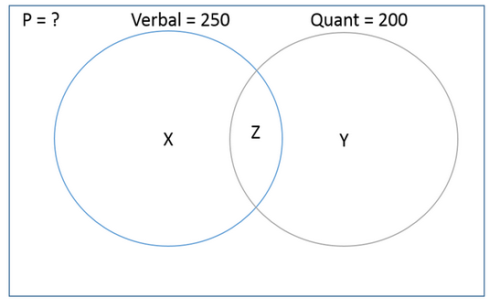We are told that

The total number of students who registered in Verbal courses = 50% of 500 =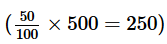Thus, X + Z = 250          ……………… (1)

Also given:

The total number of students who registered in Quant courses = 200

Thus, Y + Z = 200          ……………… (2)

Now, the sum of the numbers in the four zones of the Venn diagram will be equal to the total number of students.

That is,

X + Y + Z + P = 500      ………. (3)

We need to find the value of P.

Since we have three equations in four unknowns, we just need one more equation in these unknowns to find the value of P.

Step 3: Analyze Statement 1

30% of the aspirants registered only for Quant courses

Per this statement,

Y = 30% of 500 =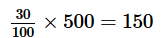………. (4)

Substituting Equations (1) and (4) in Equation (3):

250 + 150 +P = 500

P = 100

Hence, Statement 1 alone is sufficient to answer the question: What is the value of P?

(Note: We have shown the actual calculation for P here just to illustrate the calculation. In the exam, once you determine that Statement 1 provides enough information for you to solve the question, you can move to Step 4)

Step 4: Analyze Statement 2

10% of the aspirants registered for both Quant and Verbal courses

Per this statement,

Z = 10% of 500 =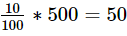By substituting this value of Z in Equations 1 and 2 respectively, we’ll be able to find the values of X and Y. And by substituting the values of X, Y and Z in Equation 3, we will be able to determine the value of P.

Thus, Statement 2 alone is sufficient to find the value of P.

Step 5: Analyze Both Statements Together (if needed)

Since Statement 1 and 2 alone are sufficient to answer the question, we don’t need this step.

QUESTION: 3

### Of the 150 people in a party, 70 were women. 30 women preferred Drink A. 50 people didn’t prefer Drink A. What was the total number of men who preferred drink A?

Solution:

As there are multiple information within the question, we need to think of a way in which we can efficiently organize this information.

We can either use a Tree structure or a Venn Diagram to solve this question.

Method 1: Tree Structure

The best way here is to use a Tree structure to organize this given information.

Let’s draw our Tree structure with the given information.

• Total number of people = 150
• Number of women = 70
• Number of women who preferred Drink A = 30
• Number of people who didn’t prefer Drink A = 50

Drawing the tree structure, we get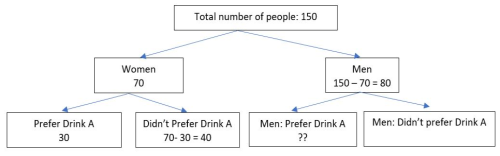Now that we have organised information in a Tree Structure, we can easily see that

• No: of men who didn’t prefer Drink A =
• No: of people who didn’t prefer Drink A (given) –  No: of women who didn’t prefer Drink A (from tree structure)

• No: of men who didn’t prefer Drink A =  50 – 40 = 10

Therefore,

Out of the 80 men, 10 men didn’t prefer Drink A.

Hence,

• Number of men who preferred Drink A = Total number of men - Number of men who didnt prefer Drink A
• Number of men who preferred Drink A = 80 – 10 = 70

Method 2: Venn Diagram

We have seen that Tree Structure provides a easy way to solve this question.

We can also use a Venn Diagram to solve this question, although this requires a bit of advanced thinking with regards to appropriate selection of sets.

We are given that there are 150 people in a party of which 70 are women and 80 are men. We are given information about the preferences of these people of a drink A.

• 30 out of 70 women prefer drink A.
• This means, 40 women don’t prefer drink A.
• Now, we have to find the number of men who prefer drink A.

Let us consider two sets: Women and Drink A.

Therefore, our Venn Diagram will look like this: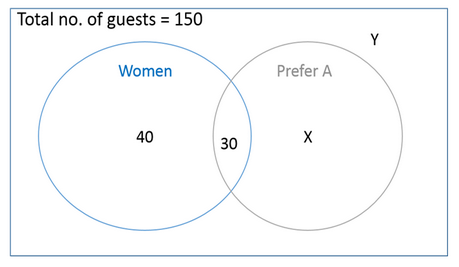In this diagram,

• X = Number of men who prefer drink A. This is the number we need to find.
• Y = Number of men who don’t prefer drink A

Now, the sum of the number of people in all the four zones will be equal to the total number of guests at the party.

Thus,

• 40 + 30 + X + Y = 150

That is,

• X + Y = 80 . . . (1)

(Basically, Equation 1 only conveys that the number of men at the party was 80)

Finding required values

We are also given that the number of people who didn’t prefer drink A is 50.

This means,

The number of people who fall outside of the gray circle (The ‘Prefer A’ circle) is 50

Thus,

• 40 + Y = 50
• Y = 10 . . . (2)

Substituting the value of Y in Equation (1) we get:

• X = 70

Thus, the number of men who prefer drink A is 70

QUESTION: 4

In a college, 300 graduates sit for the recruitment drive of three companies-A, B, and C. 120 graduates are placed in A, 180 are placed in B and 80 are placed in C. 5 students are placed in all the three companies. How many of them are not placed in any of the three companies?

(1) Out of the graduates who got placed in A, 75 and 45 are also placed in B and C respectively.

(2) Out of the graduates who got placed in C, 35 are also placed in B.

Solution:

Steps 1 & 2: Understand Question and Draw Inferences

We are given that 300 graduates from a college participate in recruitment process conducted by companies A, B, and C. The results of the process are as follows:

Let’s say:

X = the number of students who got placed in A

Y = the number of students who got placed in B

Z = the number of students who got placed in C

P = the number of students who got placed in B and C

Q = the number of students who got placed in A and C

R = the number of students who got placed in A and B

S = the number of students who got placed in all three companies

T = the number of students who were not placed in any of the companies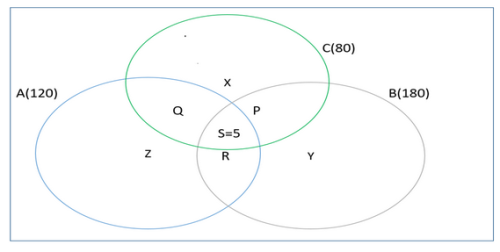Now, per the question:

X + Y + Z + P + Q + R + S + T = 300    ………… (1)

X + P + Q + S = 80      …………. (2)

Y + P + S + R = 180   …………. (3)

Z + Q + S + R = 120   …………. (4)

S = 5               …………. (5)

We need to find the value of T. However, we have 8 variables and only 5 equations. So, we need three more equations in these variables to find the value of T.

Step 3: Analyze Statement 1

Statement 1 says: Out of the number of graduates who got placed in A, 75 and 45 are also placed in B and C respectively.

Per this statement,

The number of students placed in A and B = 75

We know from the diagram that the students placed in A and B consist of R and S. So,

R + S = 75

So,  R = 70    ………….. (6)

Note that, since S represents the number of students who were placed in all the three companies, it includes the number of students who were placed in A and B.

The number of students placed in A and C = 45

We know from the diagram that the students placed in A and B consist of Q and S. So,

Q + S = 45

So,  Q = 40    ………….. (7)

Thus, we get a total of 7 equations, but we have 8 variables. So, we still can’t find the value of T.

Hence, statement I alone is not sufficient to answer the question: What is the value of T?

Step 4: Analyze Statement 2

Statement 2 says:  Out of the number of graduates who got placed in C, 35 are also placed in B.

The number of students placed in B and C = 35

We know from the diagram that the students placed in B and C consist of P and S. So,

P + S = 35

So,  P = 30    ………….. (8)

Thus, we get a total of 6 equations (5 from Steps 1 and 2, and another one in this step, from Statement 2), but we have 8 variables. So, we still can’t find the value of T.

Hence, statement II alone is not sufficient to answer the question: What is the value of T?

Step 5: Analyze Both Statements Together (if needed)

Since statement I and II alone are not sufficient to answer the question, let’s analyse both the statements together:

Now, the combination of both the statements gives us 8 equations in 8 variables, we can easily find the value of T. By plugging in the values of P, Q, and R, we get: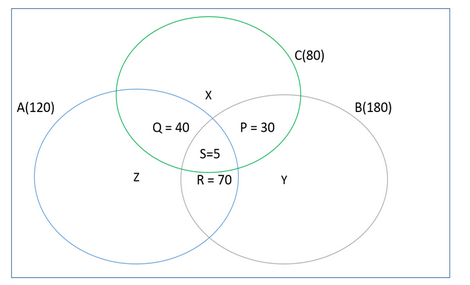Now, by plugging in the values of P, Q, R, and S in the following equations, we get:

X + Y + Z + P + Q + R + S + T = 300    ………… (1)

X + P + Q + S = 80      …………. (2)

Y + P + S + R = 180   …………. (3)

Z + Q + S + R = 120   …………. (4)

X = 5

Y = 75

Z = 5

Thus, from equation (1):

5 + 75 + 5 + 40 + 30 + 70 + 5 + T = 300

230 + T = 300

So,  T = 70

So, there are total 70 students who were not placed in any of the companies.

Both statements together are sufficient to answer the question.

(Note: We have solved for the value of T only to illustrate how such an answer could be calculated if one wanted to solve for T. The given question is a Data Sufficiency question, and therefore, you as a student need not solve for the value of T. Once you determine in Step 5 that you have 8 equations and 8 variables, and therefore the two statements together are sufficiency to find a unique value of T, you can-you should! – stop there and confidently mark the answer as C)

QUESTION: 5

100 students appeared for two tests-Physics and Chemistry. 60 students passed the Physics test and 33 students passed the Chemistry test. The school had announced a cash prize of \$1,000 for every student who passed in at least one test. What is the difference between the maximum and minimum possible values of the cash disbursal done by the school?

Solution:

Step 1: Question statement and Inferences

We are given that a total of 100 students appeared in the two tests. Out of these 100 students, 60 passed in Physics and 33 passed in Chemistry. The point to note here is that the question statement doesn’t say “60 students passed ONLY in Physics” or “33 students passed ONLY in Chemistry”. Therefore, the numbers given here are for the total number of students who passed in Physics and Chemistry respectively.

If a student passes in at least one test, the school gives him a cash prize of \$1,000. We have to find the difference in the maximum and minimum possible values of the prize money that the school disbursed to the students.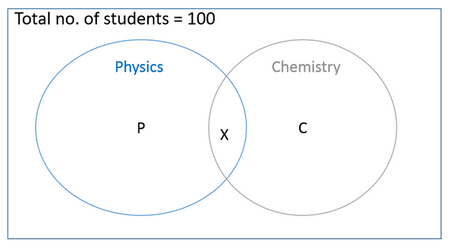Let’s say,

P = the number of students who passed only in Physics

C = the number of students who passed only in Chemistry

X = the number of students who passed both the tests

Given that,

Number of students who passed in Physics = 60

This means, P + X = 60

Or, P = 60 – X

Similarly, C + X = 33

This means, C = 33 – X

Let the number of students who qualify for money disbursement be Z.

Thus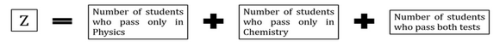Z = P + C + X

That is, Z = (60 -X) + (33 – X) + X

Z = 93 -X

As we can see, the value of Z depends on the value of X.

Case 1: Maximum value of Z

Z will be maximum when the value of X is the least. The lowest possible value of X is zero (X cannot be negative because it denotes the number of students)

Thus, Zmax = 93

Case 2: Minimum value of Z

Z will be minimum when the value of X is the maximum.

Since there are only 33 students who passed in Chemistry, X cannot exceed 33. Thus, the maximum value of X can be 33.

(Logically speaking: the number of students who passed BOTH Physics and Chemistry cannot be greater than the total number of students who passed Chemistry)

Thus, Zmin = 93 – 33 = 60

Step 2: Finding required values

Maximum possible amount of disbursement by the school = 1000* Zmax  = 1000*93 = \$93,000

Minimum possible amount of disbursement by the school = 1000* Zmin  = 1000*60 = \$60,000

Step 3: Calculating the final answer

The difference in the maximum and the minimum possible amounts of disbursement

= \$93,000 -\$60,000

= \$33,000

QUESTION: 6

Of the 120 volunteers who registered for a social welfare camp, 40 stayed for the first half of the camp duration while 10 stayed throughout the camp.  If the number of volunteers who stayed for the second half is twice the number of volunteers who did not turn up for the camp at all, how many volunteers stayed for the second half of the camp?

Solution:

Step 1: Question statement and Inferences

We are given that 120 volunteers registered for a social welfare camp. On the basis of their appearance in the first or second half of the camp, the volunteers can be divided into the following categories:

Z = the number of volunteers who stayed for only the first half

Y = the number of volunteers who stayed for only the second half

X = the number of volunteers who stayed for both the first and the second halves

T = the number of volunteers who did not show up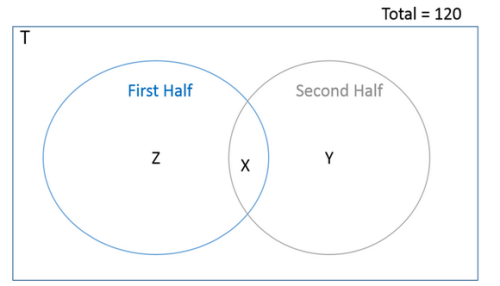Since the total number of volunteers is 120, we can write:

X + Y + Z + T = 120 . . . (1)

Also, it is given in the question that:

X = 10 . . . (2)

X + Z = 40

By substituting the value of X in the above equation, we get:

10 + Z = 40

Z = 30 . . . (3)

Also, we are given:

X + Y = 2T

Substituting the value of X in this equation, we get:

10 + Y = 2T

Or, T= (Y + 10)/2   . . . (4)

Step 2: Finding required values

Substituting Equations 2, 3 and 4 in Equation 1: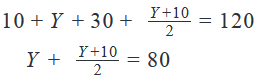2Y + Y + 10 = 160

3Y = 150

Y = 50

Step 3: Calculating the final answer

The number of volunteers who stayed for the second half = Y + 10 = 50 + 10 = 60

QUESTION: 7

In a survey conducted on women regarding their preference of cosmetics, it was found that 80% of the women surveyed were using at least one of the three brands-H & S, Dove and Pantene. 40% of those surveyed were using H & S, 30% of surveyed were using Dove and 60% of surveyed were using Pantene. 20% of the surveyed use all the three brands. What percentage of the women surveyed use more than one of the given three brands?

Solution:

Step 1: Question statement and Inferences

We are given that a survey was conducted on some women regarding their preferences of cosmetics. The results of the survey are as follows:

The percentage of women who use H&S = 40%

The percentage of women who use Pantene = 60%

The percentage of women who use Dove = 30%

Let

X = the percentage of women who use only H&S

Y = the percentage of women who use only Pantene

Z = the percentage of women who use only Dove

P = the percentage of women who use H&S and Pantene

Q = the percentage of women who use Dove and H&S

R = the percentage of women who use Dove and Pantene

S = the percentage of women who use all three products

Now, since there are only 80% women who use at least one of the three brands, there are 20% women who don’t use any of the brands.

Thus,

X + Y + Z + P + Q + R + S = 80%    ………… (1)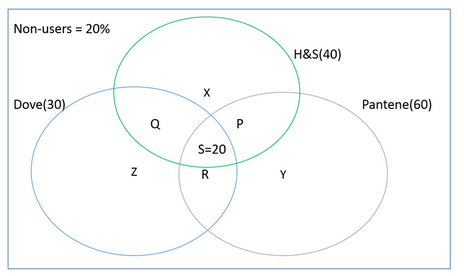Step 2: Finding required values

We can also say from the given diagram:

40% = X + P + Q + S    ………… (2)

30% = Z + Q + R + S   ………… (3)

60% = P + R + Y + S   ………… (4)

Adding equation (2), (3), and (4), we get:

130% = (X + Y + Z + P + Q + R + S) + P + Q + R + 2*S

Now, the above expression in brackets is equal to 80%              [from equation (1)]

130% = 80% + P + Q + R + 2*S

50% = P + Q + R + 2*20%

P + Q + R = 10%

Step 3: Calculating the final answer

Now, the percentage of women who use more than one brands

= the percentage of women who use two brands + the percentage of women who use three brands

= (P + Q + R) + S

= 10% + 20%

= 30%

(Note: In this question, a percentage term like 30% denotes 30% ‘of the total women surveyed’. Let the total number be t. Since the base of each percentage term used in the solution is the same – the total number of surveyed women-percentages have been added and subtracted without writing the base – as in, 30% of t – every time. If the base of each percentage term was not the same, it would have been imperative for us to specify % ‘of what’ with each term)

QUESTION: 8

A class was tested on two subjects-Mathematics and Physics. 80% of the students passed in Mathematics. This number was 120 more than the number of students who passed in Physics. If 180 students passed in both the subjects and the ratio of students who passed only in Physics and only in Mathematics was 1:7, how many students passed in neither of the two subjects?

Solution:

Step 1: Question statement and Inferences

We are given the performance of students in a class test that consists of two subjects: Physics and Mathematics. Let’s say the total number of students who appeared for the quiz be N. So, the Venn diagram for the given information can be drawn as under: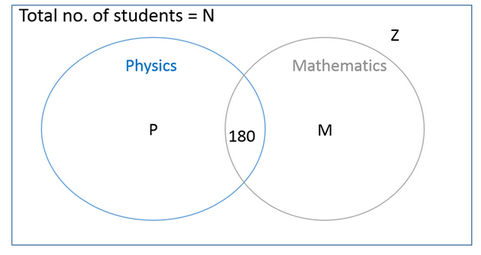Let

P = Number of students who passed only in Physics

M = Number of students who passed only in Mathematics

Z = Number of students who passed neither in Physics nor in Mathematics.

Let us now analyze the pieces of given information, one by one.

First, we are told that 80% of the students passed in Mathematics. The point to note here is that the statement doesn’t say ‘ONLY Mathematics’. Therefore, this percentage term includes students who passed only in Mathematics as well as students who passed in both Mathematics and Physics.

Therefore, we can write the equation:

M+180=80 of N

Or,  M=0.8N - 180  . . . (1)

We are also told that the number of students who passed in Mathematics was 120 more than the number of students who passed in Physics.

Again, we note that this statement doesn’t say ‘Only Mathematics’ or ‘Only Physics’. The number of students who passed in Physics will be equal to the number of students who passed only in Physics PLUS the number of students who passed in both subjects.

Thus, we can write the equation:

M + 180 = (P+180) + 120

Or, M = P + 120  . . . (2)

Finally, we are given that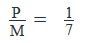Or, M = 7P . . . (3)

Step 2: Finding required values

Substituting the value of M from Equation 3 in Equation 2:

7P = P + 120

6P = 120

P = 20

From Equation 2:

M = 140

From Equation 1:

140 = 0.8N – 180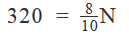N = 400

Step 3: Calculating the final answer

Now, P + 180 + M + Z = N

Substituting the values of P, M and Z in this equation, we get:

20 + 180 + 140 + Z = 400

340 + Z = 400

Z = 60

So, the number of students who failed in both the subjects is 60